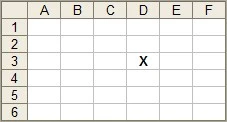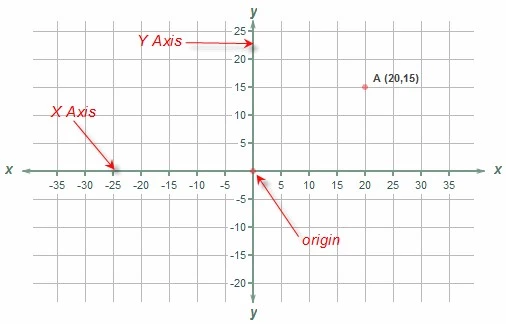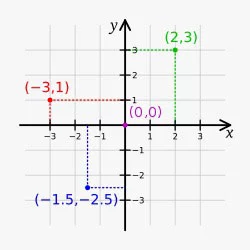Click Question to Get Free Answers >

# Coordinate Geometry NCERT Solutions : Class 9 Maths

## NCERT Solutions for Class 9 Maths : Coordinate GeometryFilter
Filters :
Classes
•
•
•
•
•
•
•
Chapters
•
•
•
•
•
•
•
•
•
•
• 6 More
Exercises
•
•
•
•

### NCERT Class 9 | COORDINATE GEOMETRY | Exercise 03 | Question No. 01

In which quadrant or on which axis do each of the points `(2,"\ "4),"\ "(3,"\ "1),"\ "(1,"\ "0),(1,2)"\ "a n d"\ "(3, 5)` lie? Verify your answer by locating them on the Cartesian plane.### NCERT Class 9 | COORDINATE GEOMETRY | Exercise 02 | Question No. 02

See Fig.3.14. and write the following: (i) The coordinates of B. (ii) The coordinates of C. (iii) The point identified by the coordinates `(3,"\ "5)` . (iv) The point identified by the coordinates `(2,"\ "4)dot` (v) The abscissa of the poi### NCERT Class 9 | COORDINATE GEOMETRY | Exercise 01 | Question No. 02

(Street Plan): A city has two main roads which cross each other at the centre of the city. These two roads are along the North–South direction and East–West direction. All the other streets of the city run parallel to these roads and are 200 m apart. There are about 5 streets in each direction. Using 1cm = 200 m, draw a model of the city on your notebook. Represent the roads/streets by single lines. There are many cross– streets in your model. A particular cross–street is made by two streets, one running in the North – South direction and another in the East – West direction. Each cross street is referred to in the following maimer : If the 2nd street running in the North – South direction and 5th in the East – West direction meet at some crossing, then we will call this cross–street (2. 5). Using this convention, find: (i)         how many cross – streets can be referred to as (4, 3). (ii)        how many cross – streets can be referred to as (3, 4).Doubtnut one of the best online education platform provides free NCERT Solutions of Maths for Class 9 Coordinate Geometry which are solved by our maths experts as per the NCERT (CBSE) guidelines. We provide the solutions as video solutions in which concepts are also explained along with answer which will help students while learning , preparing for exams and in doing homework. These Solutions will help to revise complete syllabus and score more marks in examinations.

Get here free, accurate and comprehensive NCERT Solutions for Class 9 Maths Coordinate Geometry which have been reviewed by our maths counsellors as per the latest edition following up the CBSE guidelines. We provide video solutions in which solutions to all the questions of NCERT Class 9 Maths textbook are explained in step by step and detailed way.

## The Topics of the Chapter Coordinate Geometry are :

PLOTTING OF POINTS

INTRODUCTION

RECTANGULAR AND CARTESIAN COORDINATE OF A POINT

It contains these exercises along with solved examples. All the exercises are solved in the video. Select the exercise to view the solutions exercisewise of the Chapter Coordinate Geometry:

## NCERT Solutions Class 9 Maths Chapter Coordinate Geometry Exercises:

We have covered all the exercises and also Solved examples in the videos. Along with the practise exercise Students should also practise solved examples to clear the concepts of the Coordinate Geometry. If incase you have any doubt you can watch the solutions for the given questions.Watch the solutions for the given questions in which it is also explained in the video steps to solve the questions along with answers.

# NCERT Class 9 Solutions Chapter 3 Coordinate Geometry

Class 9 is considered as one of the most difficult classes in the academic life of a student advancing towards board exams. After Class 8, the students find it difficult to cope up with the syllabus and increased levels of the new class. Students when enter Class 9, they are exposed to the new world of competition and join coaching classes for different subjects. Many students start their preparation for IIT-JEE MAINS AND ADVANCE, which sometimes makes it difficult for the students to balance between the academic studies and preparation for competitive exams. Often students find themselves lost in their preparation and stop studying after some time thinking they are not capable of learning.

It is important for every student to choose the right book so that they can easily understand the concepts and become proficient in maths. The NCERT books provided by CBSE helps students a lot in achieving high scores in exams. NCERT book for Class 9 maths gives explanation for each and every concept in the particular chapter. These explanations are equipped with mathematical tools like graphs, charts, diagrams, etc. A huge question bank is provided at the end of each topic so that students can practice and test their knowledge of the concepts just after reading the theory. The NCERT books had been a bible of mathematics for CBSE students for decades now. Every year, thousands of students benefit from the books of NCERT that makes them excel in the subject and helps them score high marks in their examinations.

NCERT books are one of the most referred books of maths that helps thousands of students achieve great scores in their exams. The book, however, does not provide a detailed solution to all the problems of the chapters covered. This issue is easily overcome by our e-learning online platform Doubtnut, which provides a detailed solution to each and every question in the book.

We at Doubtnut provide solutions for the NCERT book so that students can learn the subject easily. The solutions are presented in easy to understand manner. It is ensured that steps and methods of formulas used in the solutions are familiar to students and no such thing is there which has not been taught to students until that given chapter. Experts are always available online to solve all the queries of the learners, therefore, Doubtnut is the best platform for students to learn and practice maths in an easy and efficient way.

Doubtnut also provides a pdf format of the NCERT book Class 9 Solution so that the students can always crosscheck the concepts and methodologies used in the book. Subject matter experts and highly qualified professionals in the field of mathematics prepare the solutions. These solutions are easy to learn and grasp for better understanding of the concept. It is also made sure that the solutions given are 100% accurate. In addition, the solutions are arranged in ascending order of questions with respect to the book.

Chapter 3 of NCERT Mathematics book for Class 9 is Coordinate Geometry. The solutions for the chapter are provided stepwise representing the methods and formulas that are used to solve the problems. Each step is explained in a very simple language to ensure that students understand the basic formulas applied in that step.

## Introduction to Chapter 3 Coordinate Geometry

The chapter of Coordinate Geometry is one of the most important and easy to understand chapters of Class 9. The chapter of Coordinate Geometry is a vast topic. It is important to learn the basics of the chapter in Class 9 as coordinate geometry is also taught in higher classes so if one fails to understand the core of the chapter in this class it will be difficult for students to learn this chapter in higher classes.

### What is Coordinate Geometry?

Coordinate geometry is the branch of mathematics in which position of points on a plane is described with the help of an ordered pair of numbers.

In the chapter of coordinate geometry, students will learn topics like the Cartesian System, Coordinates of a Point, Plotting Points in the Plane and many more.

The solutions given for the book of NCERT at Doubtnut are absolutely free and can be referred any time by students. The solution is made keeping in mind that each and every student can understand with ease and follow them whenever needed.

Also, short tricks and alternative easy to use methods are provided in solutions so that students can solve problems in their examinations in less time and in a precise manner.

It is sincerely advised that students first try to attempt questions from the book with their own methods and then look for the solutions only in case when they are not able to solve on their own after several attempts. Students practicing problems in this way can find their mistakes and loopholes in their concepts. Students who are able to solve problems can also refer to the solutions for an alternative solution which will be easier and shorter. Students can and also find out their mistakes and rectify them by looking at these solutions. Learning and practicing in such manner will ensure very high scores in examinations.

## Topics and Subtopics of NCERT Mathematics book for Class 9 Chapter 3-Coordinate Geometry

The chapter of Coordinate Geometry is divided into small topics and subtopics. The topics and subtopics are divided on the basis of concepts. Each concept is listed as a topic and learning material regarding the concepts are listed as subtopics.

Each topic and subtopic are thoroughly explained in detail, giving easy to understand examples of mathematical tools as used to make the learning process more interactive for students. After the detailed explanation of concepts, it provides a question bank on the same so that students can practice the problems just after reading the theory and learn the topic completely then and there. The problems given in each chapter vary as per the requirements and the questions are arranged in order of their difficulty level. Solved and Unsolved exercises are present at the end of each chapter for revision of the concept.

Concept explanation is very detailed and illustrated with graphs, diagrams, formulas, and charts. Reading the theory given at the beginning of every chapter will help students to clear their concepts and problems provided on these concepts will support them to get comfortable with chapter further.

The way the NCERT book for class 9 is structured makes it very easy for students to learn maths in an interesting and fun way.

### What are the coordinates?

To introduce the idea, consider the grid on the right. The columns of the grid are lettered A, B, C, etc. The rows are numbered 1, 2, 3, etc. from the top. We can see that the X is in box D3, that is, column D, and row 3.

D and 3 are called the coordinates of the box. It has two parts: the row and the column. There are many boxes in each row and many boxes in each column. However, by having both we can find one single box, where the row and column intersect.The Coordinate Plane- In coordinate geometry, points are placed on the "coordinate plane" as shown below. It has two scales – one that is running across the plane is called the "x-axis" and a right angle to it called the y-axis. (These can be considered similar to the column and row as shown in the above figure). The point where the axes cross is called the origin and where both x and y are zero.

How to find a position of a point on the coordinate plane –The above-given figure can be explained as follows:

On the x-axis, the values which are on the right are positive and those to the left are negative.
On the y-axis, values above the origin are positive and those below are negative.

The location of the point on the plane is shown by two numbers; the first number tells where it is on the x-axis and the second one tells where it is on the y-axis. Together, they define a single, unique position on the plane. So, in the diagram above, point A has an x value of 20 and a y value of 15.

Ordered pair- A pair of number a and b listed in an order with ‘a’ at first position and ‘b’ at the second position is called an ordered pair. Example (2, 3) and (3, 2). Where (2, 3) ≠ (3, 2).

Mirror images- We may take x- or y-axis as a mirror of given point and then find its coordinates accordingly. Ex point is (a, b) its mirror image about x-axis will be (a, -b) and about y-axis will be (-a, b).

### Cartesian System

In the Cartesian system, the plane consists of two perpendicular axes that cross at a central point called the origin. Positions or coordinates are determined according to the east/west and north/south displacements from the origin. The east/west axis is often called the x-axis, and the north/south axis is called the y-axis. For this reason, the Cartesian plane is also known as the xy-plane. The x- and y-axes are linear number lines, meaning that each division on a given axis always represents the same increment. Points or coordinates are indicated by writing an opening parenthesis, the x value, a comma, the y value, and a closing parenthesis in that order. An example is (x,y) = (2,-5). The origin is usually, but not always, assigned the value (0, 0). With this system, we can find the position of the points on three-dimension surfaces as well.In the Cartesian coordinate system, a horizontal axis is used that is called the x-axis and a vertical axis is called the y-axis. When we will write the equations for lines in this system, it will have both the x and y variables. For example, the equation 2x + y = 2 is an example of a line in this system.  In this example, two axes cross perpendicular to each other.

## Significance of NCERT Mathematics Book for Class 9

NCERT books are one of the best books present in the market. It is a must-have book for anyone who loves maths and wants to improve and master the subject and gain high scores in their examinations. It helps the students to maintain a balance between their academic studies and competitive studies, as these days many students start their preparation for JEE from class 9th itself. It is one of the most popular books among students and helps them achieve better results.

Some features of the book of NCERT Books are:

• A detailed theory with illustrations is provided.
• A logical and algorithmic approach to every topic or concept.
• Huge question bank with illustrative examples and exercises.
• Summary of concepts and formulas at the end of each chapter.

Students must start practicing the problems from the book of NCERT Class 9 as early as possible. If done from the starting of the academic year it will be very useful. Learning maths from NCERT book will not only increase your marks, but also your interest in mathematics.

Together with NCERT books, our online platform Doubtnut has all the required study material in video-tutorials and PDF format. If students face any problem while studying from the book, they can surely switch to Doubtnut website or download its app, which will help them achieve their learning goals in an easy and convenient way possible.